# 由浅入深表达式树（一）创建表达式树 - 腾飞（Jesse) - 博客园为什么要学习表达式树？表达式树是将我们原来可以直接由代码编写的逻辑以表达式的方式存储在树状的结构里，从而可以在运行时去解析这个树，然后执行，实现动态的编辑和执行代码。LINQ to SQL就是通过把表达式树翻译成SQL来实现的，所以了解表达树有助于我们更好的理解 LINQ to SQL，同时如果你有兴趣，可以用它创造出很多有意思的东西来。

# 创建一个简单的Lambda表达式树

 1 2 3 4 5 6 `Expression> expr = x => x + 1;` `Console.WriteLine(expr.ToString());  ``// x=> (x + 1)` `// 下面的代码编译不通过` `Expression> expr2 = (x, y) => { ``return` `x + y; };` `Expression> expr3 = x => {  };`

 1 2 3 4 `internal` `Expression(Expression body, ``string` `name, ``bool` `tailCall, ReadOnlyCollection parameters)` `    ``: ``base``(``typeof``(TDelegate), name, body, tailCall, parameters)` `{` `}`

 1 2 3 4 5 6 7 8 9 10 11 12 13 `Expression> expr = x => x + 1;` `Console.WriteLine(expr.ToString());  ``// x=> (x + 1)` `var` `lambdaExpr = expr ``as` `LambdaExpression;` `Console.WriteLine(lambdaExpr.Body);   ``// (x + 1)` `Console.WriteLine(lambdaExpr.ReturnType.ToString());  ``// System.Int32` `foreach` `(``var` `parameter ``in` `lambdaExpr.Parameters)` `{` `    ``Console.WriteLine(``"Name:{0}, Type:{1}, "``,parameter.Name,parameter.Type.ToString());` `}` `//Name:x, Type:System.Int32`# 创建一个复杂的Lambda表达式树

 1 2 3 4 5 6 7 8 9 10 11 12 13 14 15 16 17 18 19 20 21 22 23 24 25 `// 下面的方法编译不能过` `/*` `Expression lambdaExpression2 = () =>` `{` `    ``for (int i = 1; i <= 10; i++)` `    ``{` `        ``Console.WriteLine("Hello");` `    ``}` `};` `*/` `       ` `// 创建 loop表达式体来包含我们想要执行的代码` `LoopExpression loop = Expression.Loop(` `    ``Expression.Call(` `        ``null``,` `        ``typeof``(Console).GetMethod(``"WriteLine"``, ``new` `Type[] { ``typeof``(``string``) }),` `        ``Expression.Constant(``"Hello"``))` `        ``);` `// 创建一个代码块表达式包含我们上面创建的loop表达式` `BlockExpression block = Expression.Block(loop);` `// 将我们上面的代码块表达式` `Expression lambdaExpression =  Expression.Lambda(block);` `lambdaExpression.Compile().Invoke();`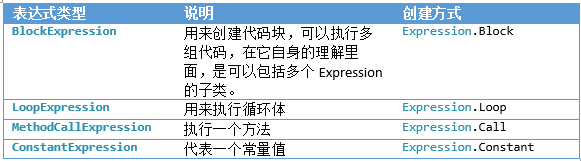1 2 3 4 5 6 7 8 9 10 11 `ParameterExpression number=Expression.Parameter(``typeof``(``int``),``"number"``);` `            ` `BlockExpression myBlock = Expression.Block(` `    ``new``[] { number },` `    ``Expression.Assign(number, Expression.Constant(2)),` `    ``Expression.AddAssign(number, Expression.Constant(6)),` `    ``Expression.DivideAssign(number, Expression.Constant(2)));` `Expression> myAction = Expression.Lambda>(myBlock);` `Console.WriteLine(myAction.Compile()());` `// 4`

 1 2 3 4 5 6 7 `LabelTarget labelBreak = Expression.Label();` `Expression.Loop(` `    ``"如果 条件 成功"` `        ``"执行成功的代码"` `    ``"否则"` `        ``Expression.Break(labelBreak) ``//跳出循环` `    ``, labelBreak);　`

 1 2 3 4 5 6 7 8 9 10 11 12 13 14 15 16 17 18 19 20 21 22 23 24 25 `LabelTarget labelBreak = Expression.Label();` `ParameterExpression loopIndex = Expression.Parameter(``typeof``(``int``), ``"index"``);` `BlockExpression block = Expression.Block(` `new``[] { loopIndex },` `// 初始化loopIndex =1` `    ``Expression.Assign(loopIndex, Expression.Constant(1)),` `    ``Expression.Loop(` `        ``Expression.IfThenElse(` `            ``// if 的判断逻辑` `            ``Expression.LessThanOrEqual(loopIndex, Expression.Constant(10)),` `            ``// 判断逻辑通过的代码` `            ``Expression.Block(` `                ``Expression.Call(` `                    ``null``,` `                    ``typeof``(Console).GetMethod(``"WriteLine"``, ``new` `Type[] { ``typeof``(``string``) }),` `                    ``Expression.Constant(``"Hello"``)),` `                ``Expression.PostIncrementAssign(loopIndex)),` `            ``// 判断不通过的代码` `            ``Expression.Break(labelBreak)` `            ``),labelBreak));` `// 将我们上面的代码块表达式` `Expression lambdaExpression =  Expression.Lambda(block);` `lambdaExpression.Compile().Invoke();`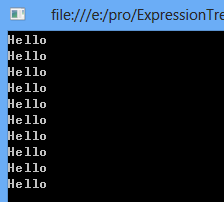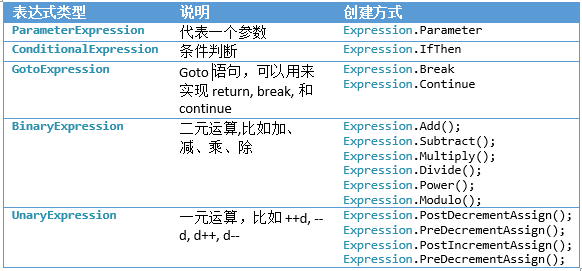• 这里的Lambda表达式实际上是一个Expression Body。
• 这个Expression Body实际上就是我们上面讲到的Expression中的一种。
• 也就是说编译器需要时间去分析你到底是哪一种？
• 最简单的x=> x+1之类的也就是Func<TValue,TKey> 是很容易分析的。
• 实际这里面允许的Expression Body只有BinaryExpression。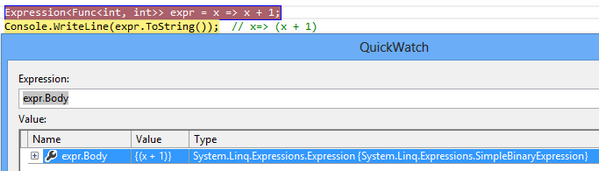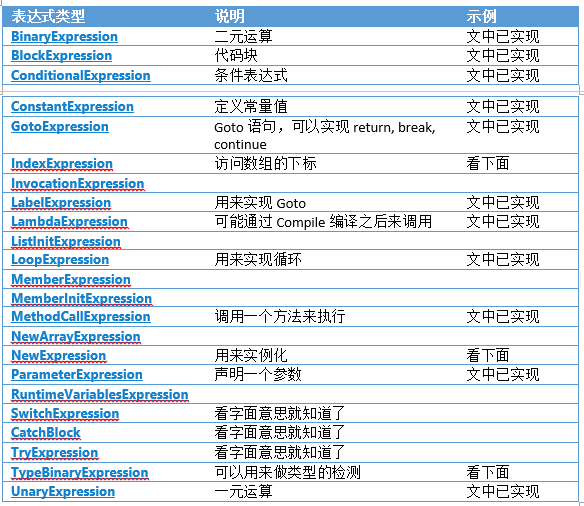### TypeBinaryExpression

 1 2 3 4 5 6 7 `TypeBinaryExpression typeBinaryExpression =` `    ``Expression.TypeIs(` `        ``Expression.Constant(``"spruce"``),` `        ``typeof``(``int``));` `Console.WriteLine(typeBinaryExpression.ToString());` `// ("spruce" Is Int32)`

### IndexExpression

 1 2 3 4 5 6 7 8 9 10 11 12 13 14 15 16 17 18 19 20 21 22 23 24 25 26 `ParameterExpression arrayExpr = Expression.Parameter(``typeof``(``int``[]), ``"Array"``);` `ParameterExpression indexExpr = Expression.Parameter(``typeof``(``int``), ``"Index"``);` `ParameterExpression valueExpr = Expression.Parameter(``typeof``(``int``), ``"Value"``);` `Expression arrayAccessExpr = Expression.ArrayAccess(` `    ``arrayExpr,` `    ``indexExpr` `);` `Expression> lambdaExpr = Expression.Lambda>(` `        ``Expression.Assign(arrayAccessExpr, Expression.Add(arrayAccessExpr, valueExpr)),` `        ``arrayExpr,` `        ``indexExpr,` `        ``valueExpr` `    ``);` `Console.WriteLine(arrayAccessExpr.ToString());` `// Array[Index]` `Console.WriteLine(lambdaExpr.ToString());` `// (Array, Index, Value) => (Array[Index] = (Array[Index] + Value))` `Console.WriteLine(lambdaExpr.Compile().Invoke(``new` `int``[] { 10, 20, 30 }, 0, 5));` `// 15`

### NewExpression

 1 2 3 `NewExpression newDictionaryExpression =Expression.New(``typeof``(Dictionary<``int``, ``string``>));` `Console.WriteLine(newDictionaryExpression.ToString());` `// new Dictionary`2()`

### InvocationExpression

 1 2 3 4 5 6 7 8 9 10 `Expression> largeSumTest =` `    ``(num1, num2) => (num1 + num2) > 1000;` `InvocationExpression invocationExpression= Expression.Invoke(` `    ``largeSumTest,` `    ``Expression.Constant(539),` `    ``Expression.Constant(281));` `Console.WriteLine(invocationExpression.ToString());` `// Invoke((num1, num2) => ((num1 + num2) > 1000),539,281)`

### 觉得文章有用就打赏一下文章作者

#### 支付宝扫一扫打赏#### 微信扫一扫打赏• QQ咨询
• 回顶